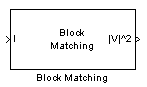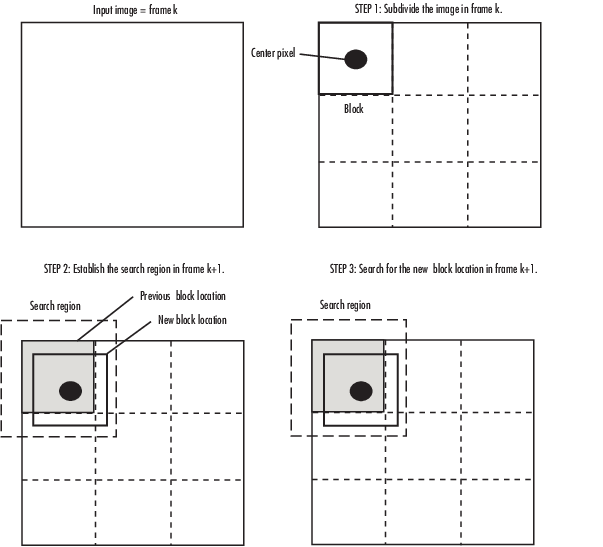Documentation

# Block Matching

Estimate motion between images or video frames

## Library

Analysis & Enhancement

`visionanalysis`## Description

The Block Matching block estimates motion between two images or two video frames using “blocks” of pixels. The Block Matching block matches the block of pixels in frame k to a block of pixels in frame k+1 by moving the block of pixels over a search region.

Suppose the input to the block is frame k. The Block Matching block performs the following steps:

1. The block subdivides this frame using the values you enter for the Block size [height width] and Overlap [r c] parameters. In the following example, the Overlap [r c] parameter is ```[0 0]```.

2. For each subdivision or block in frame k+1, the Block Matching block establishes a search region based on the value you enter for the Maximum displacement [r c] parameter.

3. The block searches for the new block location using either the `Exhaustive` or `Three-step` search method.PortOutputSupported Data TypesComplex Values Supported

I/I1

Scalar, vector, or matrix of intensity values

• Double-precision floating point

• Single-precision floating point

• Fixed point

• 8-, 16-, and 32-bit signed integer

• 8-, 16-, and 32-bit unsigned integer

No

I2

Scalar, vector, or matrix of intensity values

Same as I port

No

|V|^2

Matrix of velocity magnitudes

Same as I port

No

V

Matrix of velocity components in complex form

Same as I port

Yes

Use the Estimate motion between parameter to specify whether to estimate the motion between two images or two video frames. If you select ```Current frame and N-th frame back```, the N parameter appears in the dialog box. Enter a scalar value that represents the number of frames between the reference frame and the current frame.

Use the Search method parameter to specify how the block locates the block of pixels in frame k+1 that best matches the block of pixels in frame k.

• If you select `Exhaustive`, the block selects the location of the block of pixels in frame k+1 by moving the block over the search region 1 pixel at a time. This process is computationally expensive.

• If you select `Three-step`, the block searches for the block of pixels in frame k+1 that best matches the block of pixels in frame k using a steadily decreasing step size. The block begins with a step size approximately equal to half the maximum search range. In each step, the block compares the central point of the search region to eight search points located on the boundaries of the region and moves the central point to the search point whose values is the closest to that of the central point. The block then reduces the step size by half, and begins the process again. This option is less computationally expensive, though it might not find the optimal solution.

Use the Block matching criteria parameter to specify how the block measures the similarity of the block of pixels in frame k to the block of pixels in frame k+1. If you select ```Mean square error (MSE)```, the Block Matching block estimates the displacement of the center pixel of the block as the $\left({d}_{1},{d}_{2}\right)$ values that minimize the following MSE equation:

`$MSE\left({d}_{1},{d}_{2}\right)=\frac{1}{{N}_{1}×{N}_{2}}\sum _{\left({n}_{1},{n}_{2}\right),}\sum _{\in B}{\left[s\left({n}_{1},{n}_{2},k\right)-s\left({n}_{1}+{d}_{1},{n}_{2}+{d}_{2},k+1\right)\right]}^{2}$`

In the previous equation, B is an ${N}_{1}×{N}_{2}$ block of pixels, and s(x,y,k) denotes a pixel location at (x,y) in frame k. If you select `Mean absolute difference (MAD)`, the Block Matching block estimates the displacement of the center pixel of the block as the $\left({d}_{1},{d}_{2}\right)$ values that minimize the following MAD equation:

`$MAD\left({d}_{1},{d}_{2}\right)=\frac{1}{{N}_{1}×{N}_{2}}\sum _{\left({n}_{1},{n}_{2}\right),}\sum _{\in B}|s\left({n}_{1},{n}_{2},k\right)-s\left({n}_{1}+{d}_{1},{n}_{2}+{d}_{2},k+1\right)|$`

Use the Block size [height width] and Overlap [r c] parameters to specify how the block subdivides the input image. For a graphical description of these parameters, see the first figure in this reference page. If the Overlap [r c] parameter is not `[0 0]`, the blocks would overlap each other by the number of pixels you specify.

Use the Maximum displacement [r c] parameter to specify the maximum number of pixels any center pixel in a block of pixels might move from image to image or frame to frame. The block uses this value to determine the size of the search region.

Use the Velocity output parameter to specify the block's output. If you select `Magnitude-squared`, the block outputs the optical flow matrix where each element is of the form u2+v2. If you select ```Horizontal and vertical components in complex form```, the block outputs the optical flow matrix where each element is of the form $u+jv$. The real part of each value is the horizontal velocity component and the imaginary part of each value is the vertical velocity component.

## Fixed-Point Data Types

The following diagram shows the data types used in the Block Matching block for fixed-point signals.You can set the accumulator and output data types in the block mask as discussed in the next section.

## Parameters

Estimate motion between

Select `Two images` to estimate the motion between two images. Select ```Current frame and N-th frame back``` to estimate the motion between two video frames that are N frames apart.

N

Enter a scalar value that represents the number of frames between the reference frame and the current frame. This parameter is only visible if, for the Estimate motion between parameter, you select `Current frame and N-th frame back`.

Search method

Specify how the block searches for the block of pixels in the next image or frame. Your choices are `Exhaustive` or `Three-step`.

Block matching criteria

Specify how the block measures the similarity of the block of pixels in frame k to the block of pixels in frame k+1. Your choices are `Mean square error (MSE)` or ```Mean absolute difference (MAD)```.

Block size [height width]

Specify the size of the block of pixels.

Overlap [r c]

Specify the overlap (in pixels) of two subdivisions of the input image.

Maximum displacement [r c]

Specify the maximum number of pixels any center pixel in a block of pixels might move from image to image or frame to frame. The block uses this value to determine the size of the search region.

Velocity output

If you select `Magnitude-squared`, the block outputs the optical flow matrix where each element is of the form ${u}^{2}+{v}^{2}$. If you select ```Horizontal and vertical components in complex form```, the block outputs the optical flow matrix where each element is of the form $u+jv$.

Rounding mode

Select the rounding mode for fixed-point operations.

Overflow mode

Select the overflow mode for fixed-point operations.

Product outputAs shown previously, the output of the multiplier is placed into the product output data type and scaling. Use this parameter to specify how to designate the product output word and fraction lengths.

• When you select `Same as input`, these characteristics match those of the input to the block.

• When you select `Binary point scaling`, you can enter the word length and the fraction length of the product output, in bits.

• When you select `Slope and bias scaling`, you can enter the word length, in bits, and the slope of the product output. The bias of all signals in the Computer Vision Toolbox™ software is 0.

AccumulatorAs depicted previously, inputs to the accumulator are cast to the accumulator data type. The output of the adder remains in the accumulator data type as each element of the input is added to it. Use this parameter to specify how to designate this accumulator word and fraction lengths.

• When you select `Binary point scaling`, you can enter the word length and the fraction length of the accumulator, in bits.

• When you select `Slope and bias scaling`, you can enter the word length, in bits, and the slope of the accumulator. The bias of all signals in the Computer Vision Toolbox software is 0.

Output

Choose how to specify the word length and fraction length of the output of the block:

• When you select `Binary point scaling`, you can enter the word length of the output, in bits. The fractional length is always 0.

• When you select `Slope and bias scaling`, you can enter the word length, in bits, of the output. The bias of all signals in the Computer Vision Toolbox software is 0.

Lock data type settings against change by the fixed-point tools

Select this parameter to prevent the fixed-point tools from overriding the data types you specify on the block mask. For more information, see `fxptdlg`, a reference page on the Fixed-Point Tool in the Simulink® documentation.

## See Also

 Optical Flow Computer Vision Toolbox software

Download ebook